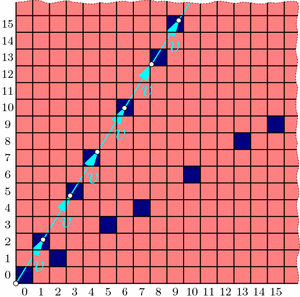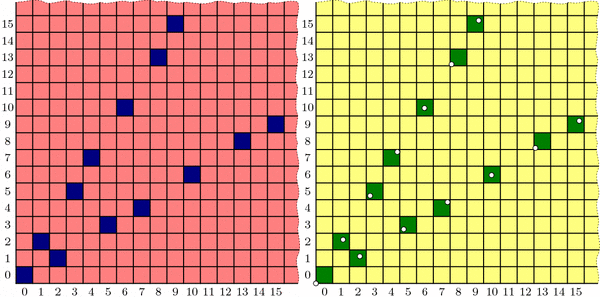# Wythoff’s Formula

Welcome back! I took a few-weeks’ blogging hiatus to focus on end-of-term craziness, but I am now resuming a regular(ish) weekly schedule throughout the summer. Let’s get back to Wythoff’s game!

Here’s a brief recap: In trying to solve for optimal play in Wythoff’s game, we saw how to algorithmically find the blue “losing positions”; we observed that these seemed to lie on two lines and, assuming this fact, we computed the lines’ slopes to be $$\phi$$ and $$1/\phi$$; and we saw how the Fibonacci numbers were hiding all over the place. But one question lingers: why lines? We’ll answer this today.

Two posts ago, we saw that if we take all of the (infinitely many) steps in the upper “V” branch and average them together, the result has slope $$\phi$$. In fact, with a little more work we can compute this “average step” exactly, not just its slope: it is the vector $$v=(\phi,\phi^2)$$. Let’s compare these average steps, namely v, 2v, 3v, etc., with the actual ones:Drawing a dot at each mutliple of the "average" step v reveals a perfect correspondence between dots and blue cells.

The dots and blue squares are perfectly matched! It seems that this may provide a precise way to easily locate all the blue cells in the upper “V” branch at once! And since the whole diagram is symmetric through the line $$y=x$$, the lower “V” branch should be governed by vector $$w=(\phi^2,\phi)$$ in the same way. Thus, a hypothesis forms:

Conjecture: The losing cells in Wythoff’s game are exactly those that contain an integer multiple of vector $$v=(\phi,\phi^2)$$ or vector $$w=(\phi^2,\phi)$$.

If we use the notation $$\lfloor x\rfloor$$ for the floor function that rounds x down to the nearest integer, then this conjecture says that the nth blue cells on the upper and lower “V” branches have coordinates $$(\lfloor n\cdot\phi\rfloor, \lfloor n\cdot\phi^2\rfloor)$$ and $$(\lfloor n\cdot\phi^2\rfloor, \lfloor n\cdot\phi\rfloor)$$ respectively. (When $$n=0$$, both formulas give (0,0).)

As we will see, this conjecture is indeed correct. How could we rigorously prove this fact?

To start, in a new grid, let’s color green all cells that fit our hypothesized formula, i.e., contain a multiple of v or w. Fill the rest of the cells with yellow. We now have two separate, a priori unrelated colorings of the grid: one with red/blue according to Wythoff’s game, and another with yellow/green according to vectors v and w.Two colorings of Wythoff's grid that we conjecture are identical. Left: the losing positions (dark blue) found previously by an iterative, optimal-play strategy. Right: multiples of vectors v and w (dotted) and their containing cells (dark green).

Proving the conjecture amounts to showing that these colorings are the same. We’ll accomplish this by showing that the yellow/green coloring behaves just like the red/blue one:

• Endgame condition: The cell (0,0) is green.
• Yellows win: From any yellow cell, it is possible to move to a green cell with one Wythoff’s game move.
• Greens lose: From a green cell, there are no other green cells accessible with a single Wythoff’s game move.

We have already observed these three properties for the red/blue coloring, and we saw that they uniquely determined the red and blue cell positions. If we could show the yellow/green coloring follows the same pattern, we could conclude that the colorings are indeed identical. So we just need to check that our yellow/green formula satisfies these three conditions!

Now that we have set the stage to dig into the meat of this proof, it is time to bid farewell until next week. See you then!

### Notes

1. Indeed, since we know that a=(1,2) and b=(2,3) appear in proportion 1 to $$\phi$$, the average step is $$\frac{1}{1+\phi}a+\frac{\phi}{1+\phi}b = (\phi,\phi^2)$$. This can also be computed from the Fibonacci observations in the most recent post. []
2. For example, $$\lfloor 2.718\rfloor = 2$$, $$\lfloor 5\rfloor = 5$$, and $$\lfloor -3.14\rfloor = -4$$. []